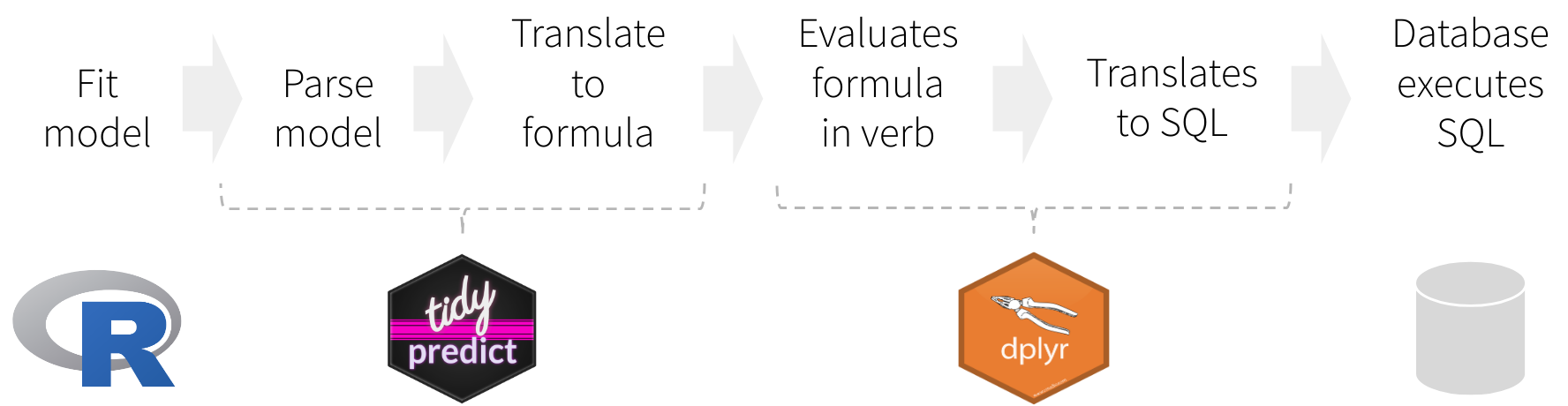# tidypredictThe main goal of `tidypredict` is to enable running predictions inside databases. It reads the model, extracts the components needed to calculate the prediction, and then creates an R formula that can be translated into SQL. In other words, it is able to parse a model such as this one:

``model <- lm(mpg ~ wt + cyl, data = mtcars)``

`tidypredict` can return a SQL statement that is ready to run inside the database. Because it uses `dplyr`’s database interface, it works with several databases back-ends, such as MS SQL:

``tidypredict_sql(model, dbplyr::simulate_mssql())``
``## <SQL> 39.6862614802529 + (`wt` * -3.19097213898374) + (`cyl` * -1.5077949682598)``

## Installation

Install `tidypredict` from CRAN using:

``# install.packages("tidypredict")``

Or install the development version using `devtools` as follows:

``````# install.packages("remotes")
# remotes::install_github("tidymodels/tidypredict")``````

## Functions

`tidypredict` has only a few functions, and it is not expected that number to grow much. The main focus at this time is to add more models to support.

Function Description
`tidypredict_fit()` Returns an R formula that calculates the prediction
`tidypredict_sql()` Returns a SQL query based on the formula from `tidypredict_fit()`
`tidypredict_to_column()` Adds a new column using the formula from `tidypredict_fit()`
`tidypredict_test()` Tests `tidyverse` predictions against the model’s native `predict()` function
`tidypredict_interval()` Same as `tidypredict_fit()` but for intervals (only works with `lm` and `glm`)
`tidypredict_sql_interval()` Same as `tidypredict_sql()` but for intervals (only works with `lm` and `glm`)
`parse_model()` Creates a list spec based on the R model
`as_parsed_model()` Prepares an object to be recognized as a parsed model

## How it worksInstead of translating directly to a SQL statement, `tidypredict` creates an R formula. That formula can then be used inside `dplyr`. The overall workflow would be as illustrated in the image above, and described here:

1. Fit the model using a base R model, or one from the packages listed in Supported Models
2. `tidypredict` reads model, and creates a list object with the necessary components to run predictions
3. `tidypredict` builds an R formula based on the list object
4. `dplyr` evaluates the formula created by `tidypredict`
5. `dplyr` translates the formula into a SQL statement, or any other interfaces.
6. The database executes the SQL statement(s) created by `dplyr`

### Parsed model spec

`tidypredict` writes and reads a spec based on a model. Instead of simply writing the R formula directly, splitting the spec from the formula adds the following capabilities:

1. No more saving models as `.rds` - Specifically for cases when the model needs to be used for predictions in a Shiny app.
2. Beyond R models - Technically, anything that can write a proper spec, can be read into `tidypredict`. It also means, that the parsed model spec can become a good alternative to using PMML.

## Supported models

The following models are supported by `tidypredict`:

• Linear Regression - `lm()`
• Generalized Linear model - `glm()`
• Random Forest models - `randomForest::randomForest()`
• Random Forest models, via `ranger` - `ranger::ranger()`
• MARS models - `earth::earth()`
• XGBoost models - `xgboost::xgb.Booster.complete()`
• Cubist models - `Cubist::cubist()`
• Tree models, via `partykit` - `partykit::ctree()`

### `parsnip`

`tidypredict` supports models fitted via the `parsnip` interface. The ones confirmed currently work in `tidypredict` are:

• `lm()` - `parsnip`: `linear_reg()` with “lm” as the engine.
• `randomForest::randomForest()` - `parsnip`: `rand_forest()` with “randomForest” as the engine.
• `ranger::ranger()` - `parsnip`: `rand_forest()` with “ranger” as the engine.
• `earth::earth()` - `parsnip`: `mars()` with “earth” as the engine.

### `broom`

The `tidy()` function from broom works with linear models parsed via `tidypredict`

``````pm <- parse_model(lm(wt ~ ., mtcars))
tidy(pm)``````
``````## # A tibble: 11 x 2
##    term        estimate
##    <chr>          <dbl>
##  1 (Intercept) -0.231
##  2 mpg         -0.0417
##  3 cyl         -0.0573
##  4 disp         0.00669
##  5 hp          -0.00323
##  6 drat        -0.0901
##  7 qsec         0.200
##  8 vs          -0.0664
##  9 am           0.0184
## 10 gear        -0.0935
## 11 carb         0.249``````

## Contributing

This project is released with a Contributor Code of Conduct. By contributing to this project, you agree to abide by its terms.